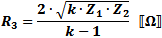# Fixed Pi and Tee Attenuators - Equations

Fixed attenuators can be designed to have either equal or unequal impedances and to provide any amount of attenuation (theoretically) equal to or greater than the configuration's minimum attenuation - depending on the ratio of Z1/Z2. Attenuators with equal terminations have a minimum attenuation of 0 dB. Unequal terminations place a lower limit on the attenuation as follows:Express in decibels as:In the attenuator formulas below:, which is the linear attenuation ratio of the two powers or voltages (note that "k" has a minimum value if Z1 and Z2.are not equal).

If, as is typical, the attenuation is given in decibels (K dB vs. k), then convert to a ratio as follows:<———>An online attenuator calculator is provided at the bottom of the page.

 Unbalanced Tee (T) Attenuator These equations apply to the two forms of Tee attenuators at the left.If  Z1 = Z2, then:Balanced Tee (T) AttenuatorUnbalanced Pi (π) Attenuator These equations apply to the two forms of Pi attenuators at the left.If  Z1 = Z2, then:Balanced Pi (π) AttenuatorNote: Only enter values in the yellow cells or risk overwriting formulas!

Input Resistance: Output Resistance:

Attenuation: dB

 k = (Pin/Pout)

Tee Attenuator       Pi Attenuator
Ω

RinRout

RinRout
Ω

An RF Cafe visitor wrote to say that he thought the above equations might be in error when unequal source and load termination resistances are used. The image below shows the mathematical steps that prove the equations are correct. It uses a source resistance of 50 ohms and a load resistance of 100 ohms, with an attenuation of 10 dB. Resistor values for both the "T" and ""Pi" attenuators were determined using the attenuator calculator on RF Cafe (which uses these equations).About RF CafeCopyright: 1996 - 2024Webmaster:    Kirt Blattenberger,    BSEE - KB3UON RF Cafe began life in 1996 as "RF Tools" in an AOL screen name web space totaling 2 MB. Its primary purpose was to provide me with ready access to commonly needed formulas and reference material while performing my work as an RF system and circuit design engineer. The Internet was still largely an unknown entity at the time and not much was available in the form of WYSIWYG ... All trademarks, copyrights, patents, and other rights of ownership to images and text used on the RF Cafe website are hereby acknowledged. My Hobby Website:  AirplanesAndRockets.com